# Recognition of Intrusive Alphabets to the Arabic Language Using a Deep Morphological Gradient

Recognition of Intrusive Alphabets to the Arabic Language Using a Deep Morphological Gradient
Research Team Complex Systems and Interactions, Faculty of Applied Sciences, Iben Zoher University, Ait Melloul-Agadir 80000, Morocco

Corresponding Author Email:
mouhssine.elatillah@edu.uiz.ac.ma
Page:
277-284
|
DOI:
https://doi.org/10.18280/ria.340305
15 February 2020
|
Accepted:
26 May 2020
|
Published:
30 June 2020
| Citation

OPEN ACCESS

Abstract:

The optical character recognition field was one of the key areas of evidence for deep learning methods and has become one of the most successful applications of this technology. Despite that the Arabic is among the most spoken languages in the world today. the optical recognition of Arabic manuscript characters by the algorithms of deep learning remains insufficient. Recently, some studies are moving towards this side and give remarkable results either for the recognition of alphabets or Arabic numbers. We present a deep architecture to solve the problem of the recognition of intrusive handwritten characters to Arabic language. We use a fusion between the morphological gradient method to detect the contours of the alphabets, and multi-layer perceptron (MLP) network with regularization parameters like batch normalization. We apply this model for a database that we created. The classification accuracy was 100% with a very small loss of 0.2%.

Keywords:

deep learning, multilayer perceptron (MLP), morphological gradient, optical character recognition

1. Introduction

Characters Recognition is one of the difficulties  to be addressed by the field of artificial intelligence until today. It is the Optical Character Recognition (OCR) field that designates computer processes for the transformation of images of printed texts, typewritten, or handwritten in text files. Overall, the handwritten recognition systems are two: online and offline systems . The capacity to process large quantities of script data in specific contexts will be important. The OCR is interested in old documents through the transcription automation of their textual contents, given the complex and irregular nature of writing . The recognition of Arabic texts is developing slowly compared to other languages . Despite the fact that Arabic is the main language of the North of Africa and the Middle East. It is widely spoken in many other countries. Statistically, Arabic is one of the top five languages spoken in the world today [5, 6]. In the last two years, the recognition of Arabic alphabets by deep learning algorithms, as well as Arabic numerals , is a remarkable development by Arabian researchers. The strong point of the deep learning compared to the classical algorithms of artificial intelligence is the extraction of the features of the images by the algorithm itself using filters at the beginning of the process of training. Whereas the classical algorithms are based on vectors of features extracted by experts (searchers, programmers). So, as long as the images are clear and show the details of its objects, the results of the deep learning algorithms will be satisfying. Most of the studies in this area have focused on predefined deep learning modeling, especially the convolutional neural network (CNN) . This is the case for the study  which uses the CNN model with regularization parameters like batch normalization to avoid oversampling. The model is applied on both databases, AIA9k and AHCD. The classification accuracies for the two datasets were 94.8% and 97.6% respectively. Another work model CNN has formed and tested a dataset of 16,800 Arabic characters in which the optimization methods implemented to increase the performance of CNN. The most common machine learning methods typically apply a combination of feature extractor and classifier that can be trained. The use of convolutional neural network leads to huge enhancements on various machine learning classification algorithms. This CNN proposal gives an average loss of 5.1% on the test data . The use of a CNN model with modifications to its parameters to be suitable with the treated problem is able to give satisfying results even for more complete databases (colored images). This is the case of the studies that have been quoted beforehand. While the images processed by the ROC domain are in general binary or gray-scale images, this nature can process by classic classification algorithms (SVM, linear regression ...) and gives remarkable results better than some models of deep learning, like the study  which combines three classical algorithms: KNN (K-Nearest Neighbors) classifier, SVM (support vector machine), and RF (Random Forest) classifier . These methods give successively 99.71%, 97.88%, and 99.91% as precision for the MNIST database.

The CNNs mentioned above are very solid architectures at the level of deep learning in general because they are based on convolutional layers. These layers have the role of extractors of the images’ features through filters and pooling layers followed by fully connected layers (like the structure of MLP that we used in this paper). The advantage of this structure is the neglect of human intervention which is limited to choosing the general confederations of CNN (number of layers, filters and neurons per layer, the activation functions used. etc.) and they change several times to get the best accuracy possible. This negligence can be considered a weak point sometimes despite the roughness of the CNNs because they based on deep architecture with complex mathematical functions with thousands of parameters which is out of control for human beings. For these reasons, we will focus in this study on the database itself through the clarity of the objects of its images to overcome the weak points of deep learning methods cited above. We will use a combination of two methods of machine learning. The first is the morphological gradient to illuminate the processed alphabet through the detection of its contours, followed by an MLP which is a deep network. Our model is applied to a database of Arabic intruder alphabets (gaf, ve, pe).

2. Method

2.1 Motivation

The objective of this study is to treat the intrusive alphabets to the Arabic language which is not treated previously at the level of the recognition of the Arabic characters. All studies in this side focused on Arabic characters and numbers [7, 9, 13]. The alphabets treated by this study are similar to few Arabic alphabets with a difference in the number of points below or above the alphabet. This similarity shown in Table 1 requires concentration on the points that consist each alphabet for a deep learning algorithm to be able to distinguish one to the other

2.2 Database creation

The supervised recognition of images by deep learning algorithms consists of the process of training that requires a database of images related to the processed alphabets. This database must contain maximum images with different shapes of alphabets treated. Because of the absence of a database to use, it is created, taking into consideration the different possibilities of writing processed alphabets. To ensure the efficiency of the images created, manuscripts of people of different ages have been captured. Each image size is 64x64 pixels. In general, Arabic alphabets are re-formed according to their position in the words as you see in Table 2.

Table 1. The similarity and the differentiation between the original alphabets and intruders to the Arabic language

Name

Gaf

Pe

Ve

Intruder

alphabet

## 0.png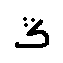## 00.png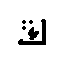## 000.png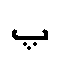## 0000.pngOriginal

alphabet

## 1.png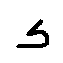## 11.png## 111.png## 1111.png## 11111.png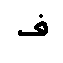Similitude

The same basic form

Difference

Three points above the intruder

alphabet

Three points below the intruder alphabet instead of one for the original alphabet

Three points above the intruder

alphabet instead of one or two for

the original alphabets

Table 2. Main forms of intruder characters to the Arabic language

Name

isolated

Initial

Median

Final

Gaf

## 2.png## 22.png## 3.png-

## 3333.png-

## f.png## ff.png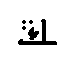Pe

## 222.png## 33.png## 33333.png## fff.pngVe

## 2222.png## 333.png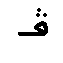## 3333333.png## ffff.pngThe database consists of 1200 images of handwritten alphabets intrusive to the Arabic language divided into: 450 characters for the Gaf alphabet, 389 for Pe, and 361 for Ve as shown in Figure 1.

## 1.jpgFigure 1. Number of alphabets per class

In order to build a solid database we are trying to capture a suitable number of images per class based on exiting databases such as Arabic Handwritten characters Dataset which contains 600 images per class. The images in our database are binary images (white / black images) with a uniform size of 64x64 pixels. The alphabets are generally centered in the middle of the images for this we have reduced their size to 24x24 pixels before processing. This redimensioning which is used in most of the image recognition algorithms is capable of removing certain images’ features. This is why we are testing several forms in order to preserve the images’ features. The Figure 2 shows samples of our database.

## 2.jpgFigure 2. Samples of our database

## 3.jpg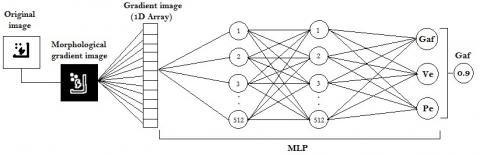Figure 3. Deep morphological gradient method

2.3 Architecture

We discuss in this section our method and its ability to improve the recognition accuracy of the processed dataset. For this purpose, we test our database with a multilayer perceptron (MLP) of three layers (input, output and an intermediate or hidden layer). This MLP has already used for the recognition of Arabic numbers . This is preceded by the binary threshold method for Segmenting images based on the fact that these are binary images. The first fully connected layer receives the image pixels densities vector. Its size is 576 (we resize the images to 24x24 since the alphabets are centered in the middle of the images). The second fully connected layer as the first one consists of 512 neurons with the function of activation ReLU in order to eliminate the negative values for output values of each neuron. The third layer consist of 3 neurons is linked to the target classes (the three alphabets) with SoftMax as an activation function for each neuron to render the results as categorical probabilities between 0 and 1 taking a predicate for a class of the three classes (the alphabets treated) is true. The Adam Optimizer is used during the training process to get the best model possible.

Our method (deep morphological gradient) shown in The Figure 3, is very close to the first method except that the morphological gradient method is used instead of binary threshold. The gradient algorithm uses the inverse binary threshold method, followed by the difference between the two morphological methods, dilation and erosion in order to detect the contours of the processed images. Dilation enlarges the body of the alphabet (i.e. white part of the image). We use an array of 3x3 dimension containing ones as kernel. In the same way, the erosion corrodes white part of the image. The difference between them gives us a clear alphabet outline. Table 3 shows the morphological gradient of some alphabets.

Table 3. Morphological gradient of some alphabets

Original image

## 0.png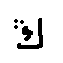## 1.png## 2.png## 3.png## 4.png## a.jpg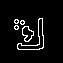## b.jpg## c.jpg## d.jpg## e.jpg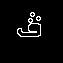We know that a deep learning algorithm goes through two main stages (Forward and Backward) to get the best parameters (weights and biases) to build the final model. Below, the two-step process for our proposed method:

The process started with a morphological gradient algorithm as follows:

G(Ig) = (Ig ⊕ B) – (Ig ⊖ B)  (1)

where, Ig: $E \rightarrow R$ a grayscale image, E a discrete grid E (like R2 or Z2), and B a structuring element in grayscale, ⊕ and ⊖ respectively denote the dilatation and erosion [15, 16].

2.3.1 Forward

The Forward path is essentially a set of operations that transform the network input into an exit space. During the inference phase, the neural network is based solely on the forward passage. We assume here that each neuron, except the neurons of the last layers, uses the ReLU activation function (the last layer uses SoftMax). Activation functions are used to introduce non-linearity into the system, which allows learning of complex functions. So, these are the calculations done in the first layer:

For neuron 1 of the first layer:

$\mathrm{h}^{\mathrm{in}}=\sum_{i=1}^{576} \mathrm{W}_{\mathrm{i}} \mathrm{G}\left(\mathrm{x}_{\mathrm{i}}\right)+\mathrm{b}_{1}$  (2)

$\mathrm{h}^{\text {out }}=\operatorname{Relu}\left(\sum_{i=1}^{576} W_{i} G\left(x_{i}\right)+b_{1}\right)$  (3)

By rewriting this as a matrix for all the neurons of the first layer, we have:

$\mathrm{h}^{\mathrm{in}}=\left[\begin{array}{ccc}x_{1} \ldots x_{576}\end{array}\right] \times\left[\begin{array}{ccc}w_{1}^{1} & \cdots & w_{1}^{512} \\ \vdots & \ddots & \vdots \\ w_{576}^{1} & \cdots & w_{576}^{512}\end{array}\right]+\left[b_{1} \ldots b_{512}\right]$ (4)

Now, if we represent inputs in the form of matrix I, weights of neurons equal to W and bias to B, we obtain:

$h^{\operatorname{in}}=I \times W+B$  (5)

$\mathrm{h}^{\text {out }}=\operatorname{Relu}\left(h^{i n}\right)$  (6)

This can be generalized for any layer of a fully connected neurals network such as

$h_{0}^{\text {out}}=I$  (7)

$h_{i}^{i n}=h_{i-1}^{\text {out}} \times W_{i}+B_{i}$  (8)

$h_{i}^{\text {out}}=F_{i}\left(h_{i}^{i n}\right)$  (9)

where, i is the layer number and F is the activation function for a given layer. Applying this formula to each layer of the network, we will implement the forward pass and get the network output.

2.3.2 Backward (Backpropagation)

In order to measure the degree of inconsistency of our predicates compared to real labels, we need a metric. This metric is called the loss function, which measures the performance of the model. This is a positive value that decreases as the network becomes more consistent with its predicates. The Cross-Entropy is used as loss function for our model. It is defined as follows:

$C E=-\sum_{j}^{n} y_{j} \times \ln \left(\hat{y}_{j}\right)$  (10)

where, $\hat{y}$  is the vector of the network output, y the vector of the true labels, and n is the number of classes. Now, in order to find the error gradients with respect to each variable, we start with the last layer and taking a partial derivative of the loss versus the weight of the neurons, we get:

$\frac{\partial C E}{\partial W_{3}}=\frac{\partial C E}{\partial h_{3}^{i n}} \times \frac{\partial h_{3}^{i n}}{\partial W_{3}}$  (11)

Knowing that in case of softmax activation function and cross-entropy loss, we have:

$\frac{\partial C E}{\partial h_{3}^{i n}}=\hat{y}-y$  (12)

Now we can find the gradient for the last layer like:

$\frac{\partial C E}{\partial W_{3}}=(\hat{y}-y) \frac{\partial h_{3}^{i n}}{\partial W_{3}}$

$=(\hat{y}-y) \frac{h_{3}^{\text {out}} W_{3}+B_{3}}{\partial W_{3}}$

$\quad=\left(h_{2}^{\text {out}}\right)^{T}(\hat{y}-y)$

$\frac{\partial C E}{\partial W_{3}}=\left(h_{2}^{\text {out}}\right)^{T} \delta_{3}$  (13)

where, $\delta_{3}=(\hat{y}-y)$

By proceeding with layers 2 and 1, we successively found:

$\frac{\partial C E}{\partial W_{2}}=\left(h_{1}^{\text {out}}\right)^{T} \delta_{2}$  (14)

$\frac{\partial C E}{\partial W_{1}}=\left(h_{0}^{o u t}\right)^{T} \delta_{1}$  (15)

Following the same procedure for bias:

\begin{aligned} \frac{\partial C E}{\partial B_{3}}=(\hat{y}-y) \frac{\partial h_{3}^{i n}}{\partial B_{3}} &=(\hat{y}-y) \frac{h_{3}^{o u t} W_{3}+B_{3}}{\partial B_{3}} \\ &=(\hat{y}-y)=\delta_{3} \end{aligned}  (16)

By proceeding with layers 2 and 1, we find successively δ2 and δ1. We can now follow a common pattern, which can be generalized as follows:

$\frac{\partial C E}{\partial B_{i}}=\delta_{i}$  (17)

With these equations, we can calculate the error gradient as a function of each weights / biases. To reduce the error, we need to update our weights / biases in a direction opposite to the slope. This idea is used in the Adam algorithm, and is defined as follows:

$m_{t+1}=\beta_{1} m_{t}+\left(1-\beta_{1}\right) \frac{\partial C E_{t}}{\partial x_{t}}$  (18)

$v_{t+1}=\beta_{2} v_{t}+\left(1-\beta_{2}\right) \frac{\partial C E_{t}^{2}}{\partial x_{t}}$  (19)

mt is the estimate of the first moment (the average), and vt is the estimate of the second moment (the non-centered variance) of the gradients, hence the name of the method. Since they are initialized as vectors of 0, the authors of Adam observe that they are biased towards zero, during the initial time steps, and especially when the decay rates are low (that is to say $\beta_{1}$  and $\beta_{2}$ are close to 1).  xt takes the two variables ( wt or bt ). They neutralize these biases by calculating the first and the second moment estimates of biases:

$\widehat{m}_{t}=\frac{m_{t}}{1-\beta_{1}^{t}}$ And $\hat{v}_{t}=\frac{v_{t}}{1-\beta_{2}^{t}}$  (20)

Then, we get the Adam update equation:

$x_{t+1}=x_{t}-\alpha \frac{\widehat{m}_{t}}{\sqrt{\hat{v}_{t}}+\varepsilon}$  (21)

where, x is a variable that can be dragged (W or B), t is the current step (iteration of the algorithm) and α is a learning rate. Now, set $\varepsilon$ = 108, α = 0.001 (small values imply a longer formation process, whereas high values lead to an unstable formation process), β1 = 0:9 and β2 = 0:999.

The erosion and dilation are the two elementary operators of mathematical morphology. They are noted respectively Ig ⊖ B and Ig ⊕ B, where Ig corresponds to the binary image to be processed and B the structuring element . We define these operators mathematically as follows:

\begin{aligned} \operatorname{Ig} \oplus & \mathrm{B}=\mathrm{U}_{\mathrm{b} \in \mathrm{B}} \mathrm{Ig}_{\mathrm{b}} \\ &=\mathrm{U}_{\mathrm{x} \in \mathrm{Ig}} \mathrm{B}_{\mathrm{x}} \\ &=\{\mathrm{x}+\mathrm{b}, \mathrm{x} \in \mathrm{B}, \mathrm{b} \in \mathrm{B}\} \end{aligned}  (22)

\begin{aligned} \operatorname{Ig} \ominus \mathrm{B} &=\cap_{b \in B} \lg -\mathrm{b} \\ &=\{\mathrm{p} \in \mathrm{E}, \mathrm{Bp} \subseteq \mathrm{Ig}\} \end{aligned}  (23)

A morphological dilation consists in moving the structuring element on each pixel of the image, and to see if the structuring element "touches" (or more formally intersects) the structure of interest. The result is a structure that is larger than the original structure. Depending on the size of the structuring element, certain particles may be connected, and certain holes may disappear. Conversely, Erosion is the opposite operation, which is defined as a dilation of the complementary of the structure. It consists in searching for all the pixels for which the structuring element centered on this pixel touches the outside of the structure. The result is a cropped structure. We observe the disappearance of particles smaller than the structuring element used, and the possible separation of large particles. The gradient is the difference between dilation and erosion of an image . The result will appear as an outline of the image.

2.3.4. Multilayer perceptron

An MLP (Multilayer Perceptron) is a type of formal neural network that is organized into several layers. Information flows from the input layer to the output layer [19, 20]. It is used to classify groups that are not linearly separable. The Figure 4 shows an MLP with a hidden layer.

## 4.jpgFigure 4. MLP with a hidden layer

Each MLP node (neuron) calculates the weighted sum of the inputs. This sum passes as a parameter to a transfer function which will calculate the value of the state of the neuron that is referred to as an activation function. In our network, we use two activation functions. The ReLU function in the input and the hidden layers. The Softmax function in the output layer in order to give us the class prediction.

(1) Rectified Linear Units

These are the most popular functions these days. They allow faster training compared to the sigmoid and tanh functions, being lighter. Widely used for CNN, RBM, and multi perceptron networks. The input of this function is always greater than zero (i.e. belongs to R+) .

$\sigma\left(Y_{i}^{l}\right)=\max \left(0, Y_{i}^{l}\right)$  (24)

Because of its simplicity and non-linearity, ReLU is the most used activation function. Its principle is to get positive input. As well as its derivative is a constant function equal to 1. The speed of the models is among the most important creditors, especially when we talk about large networks, research has shown that ReLUs are the most efficient in this area. The frameworks TensorFlow  and TFLearn make it easy to use ReLU on hidden layers .

(2) Softmax function

Softmax assigns decimal probabilities to each class of a problem to several classes. The sum of these decimal probabilities must be equal to 1. This additional constraint allows learning to converge more quickly than it would otherwise. SoftMax indicates the probability that one of the classes is true using a categorical probability distribution. SoftMax is implemented via a neural network layer just before the result layer. The SoftMax layer must have the same number of nodes as the result layer. It is writing mathematically as:

$\sigma(z)_{j}=\frac{e^{z_{j}}}{\sum_{k=1}^{K} e^{z_{k}}}$  (25)

It takes as input a vector $\mathrm{z}=\left(\mathrm{z}_{1}, \ldots, \mathrm{z}_{\mathrm{k}}\right)$ of K real numbers and a vector $\sigma(z)$ of K strictly positive real numbers and of sum 1.

In the backpropagation phase, we need to use an optimization function in order to update the network parameters (weights and bais). We used one of the newest and most efficient algorithms which is ADAM algorithm.

There are many possible improvements to the classic stochastic gradient descent; we use the ADAM algorithm which estimates the average, and the variance of the gradient for each parameter geometrically decreasing with respect to time. There is also a momentum which adds inertia to the update. Thanks to these estimates, the updating of the parameters is smoother and the variance is reduced. However, in this algorithm, it is necessary to define an overall learning rate α [23, 24].

3. Experimental Environment and Experimental Conditions

The two proposed models are tested against a database of 1200 images and validated against the samples of 200 images of data. The number of parameters that make up our model is 559619. We use the Tensoflow and Keras libraries  to implement our source code using the python programming language. The supervised training of proposed method is carried out with 1000 training samples, and then validated with 200 test samples. The working material is a computer of the following configuration: Intel CPU i3-3120M Processor (3M Cache, 2.50 GHz) and 4 GB of RAM.

The first method gives unsatisfactory results. The accuracy of the training dataset is 85.8% which devalues this model compared to other optical character recognition (OCR) models. The validation samples give acceptable results that tend towards 84.6%. That shows the limitation of knowledge of the new images outside the training dataset. The loss of data during the execution process is remarkable, and tends to 31.5% for the training data. The Figure 5 shows the Accuracy / loss with regard to the number of iterations performed for the two databases (training and validation).

Our model gives exceptionally successful outcomes than the first. The training dataset accuracy starts with a high value equal to 78%, the same for the validation base which starts with 93% precision. These values are due to the morphological gradient method which we added before the MLP to highlight the bodies of the alphabets through the detection of their contours. We use the same number of iterations as the first method to show the preference of our method. The accuracies of the two databases are incremented during the course of the algorithm until reaching 100% and 96% of the training and loss base successively. The loss of data during the execution process is too low at 0.2% for the training data. It starts with a small value (45%) comparing to the first method which starts with 100% of loss.

## 51.png（a）Model accuracy

## 52.png(b) Model loss

Figure 5. The variation of accuracy and loss for Deep threshold method

These results are given based on:

1. Morphological gradient which increases the detection capacity of the alphabets constituting the images of our database. It helps the perceptrons of the first layer to detect pixels of high densities. The backpropagation plays the most important role in the process of regulating the biases and weights of the perceptrons with the aim of activating some and deactivating the rest.
2. The Relu activation function which preserves the densities of positive pixels and replaces any negative input value with 0. And for its gradient, it becomes zero for negative values and is worth 1 for positive values. This property is very interesting in the learning phase because it avoids and corrects the problem of vanishing gradient [26, 27].

The Figure 6 shows the variation of precision / loss with respect to the number of iterations performed for the two databases (training and validation). The Table 4 shows the difference between the two methods proposed in terms of precision and loss of data.

## 61.png(a) Model accuracy

## 62.png(b) Model loss

Figure 6. The variation of accuracy loss for the deep morphological gradient method

## 71.png(a) Arabic handwritten digits

## 72.png(b) Arabic handwritten characters

## 73.png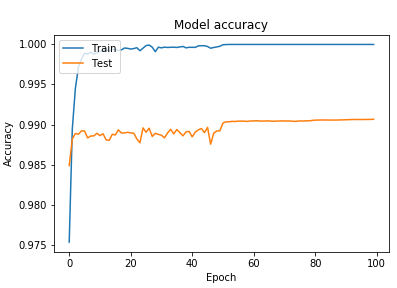(c) MNIST

Figure 7. The accuracies of datasets

Table 4. Comparison between the performances of the proposed methods

 Method Accuracy (%) Loss (%) Deep threshold 85.8 31.5 Deep morphological gradient 100 0.2

Table 5. Results obtained by the application of our method on the four databases quoted in our study

 Dataset Number of images Accuracy (%) Loss (%) Arabic Handwritten Digits 60,000 99.9 0.09 Arabic Handwritten characters 16,800 99.9 0.32 MNIST 60,000 100 10-5 Our Dataset 1,200 100 0.2
To ensure the effectiveness of our proposed method, we tested it against three other databases: Arabic Handwritten Digits Dataset , Arabic Handwritten characters Dataset  and the MNIST database of handwritten digits . The results obtained are ideal for the three databases. The accuracies were successively 99.9%, 99.9% and 100% for the three databases. The results obtained show the robustness of our method for the recognition of manuscripts in general. For the three databases the algorithm started with very high values from the first iteration (more than 97%). The Figure 7 shows the variation of accuracy of each database in relation to the number of model iterations. The Table 5 shows the accuracies and the losses obtained by the application of our method on the four databases quoted in our study.
4. Conclusion and Perspectives

The industrial field and the government sectors use some automated software systems which are based on the OCR models. Therefore, the recognition of intrusive Arabic alphabets could enrich these systems. In this study, we present a deep learning system based on the morphological gradient, followed by a multilayers neuron network (MLP) capable of classifying intrusive handwritten characters to the Arabic language with 100% classification accuracy for our database.

We implement our model using python language, Keras and Tensorflow frameworks. As future work, in addition to working more with deep learning, we plan to test a larger and more diverse database. We can do that by merging several database sources that aim at recognizing characters, numbers, and characters intruding to the Arabic language at the same time. We plan to set up a deeper network that is able to recognize a database of 41 classes (28 Arabic characters, 10 Arabic numbers and 3 intruder characters to the Arabic language).

Nomenclature
 G(Ig) gradien of image I Ig ⊕ B the dilation of image x per the structuring element B Ig ⊖ B the erosion of image x per the structuring element B hiin the input matrix of a layer i hiout the output matrix of a layer i CE function cross-entropy mt estimate of the first moment (the average) of the gradients vt estimate of the second moment (the non-centered variance) of the gradients $\sigma\left(Y_{i}^{l}\right)$ rectified linear units function (ReLUs) $\sigma(z)_{j}$ softmax function
References

 Ashiquzzaman, A., Kawsar, T.A. (2018). KERTAS: Dataset for automatic dating of ancient Arabic manuscripts. International Journal on Document Analysis and Recognition (IJDAR), 21: 283-290. https://doi.org/10.1007/s10032-018-0312-3

 Plamondon, R. (2000). On-line and off-line handwriting recognition: A comprehensive survey. IEEE Transactions on Pattern Analysis and Machine Intelligence, 2(1): 63-84. https://doi.org/10.1109/34.824821

 Volker, M., Haikal E.A. (2011). Guide to OCR for Arabic. Springer-Verlag, Berlin.

 Abandah, G., Khedher, M., Younis, K. (2008). c. The 5th IASTED International Conference on Signal, Innsbruck, 22: 128-133.

 Das, N., Mollah, A.F., Saha, S., Haque, S.S. (2006). Handwritten Arabic numeral recognition using a multi layer percepron. National Conference on Recent Trends in Information Systems, pp. 200-203.

 Abdleazeem, S., El-Sherif, E. (2008). Arabic handwritten digit recognition. International Journal of Document Analysis and Recognition (IJDAR), 11: 127-141. https://doi.org/10.1007/s10032-008-0073-5

 Ahmed, E.S., Hazem, E.B., Mohamed, L. (2016). CNN for handwritten Arabic digits recognition based on LeNet-5. The International Conference on Advanced Intelligent Systems and Informatics, pp. 566-575. https://doi.org/10.1007/978-3-319-48308-5_54

 Yiğit, A., Işik, Z. (2020). Applying deep learning models to structural MRI for stage prediction of Alzheimer’s disease. Turkish Journal of Electrical Engineering & Computer Sciences, 28: 196-210. https://doi.org/10.3906/elk-1904-172

 Younis, K.S. (2017). Arabic handwritten character recognition based on deep convolutional neural networks. Jordanian Journal of Computers and Information Technology (JJCIT), 3(3).

 Ahmed E.S., Mohamed, L., Hazem, E.B. (2017). Arabic handwritten characters recognition using convolutional neural network. WSEAS Transactions on Computer Research, 5: 11-19.

 Anuj, D., Aashi, D. (2017). Handwritten digit recognition using deep learning. International Journal of Advanced Research in Computer Engineering & Technology (IJARCET), 6(7): 990-997.

 Nudrat, N., Muhammad, H.Y., Aun, I., Sergio, A.V. (2020). Deep temporal motion descriptor (DTMD) for human action recognition. Turkish Journal of Electrical Engineering & Computer Sciences, 28: 1371-1385. https://doi.org/10.3906/elk-1907-214

 Ahmed, E.S., Mohamed, L., Hazem, E.B. (2017). Arabic handwritten characters recognition using convolutional neural network. WSEAS Trasctions on Computer Research, 7: 11-19.

 Ashiquzzaman, A., Tushar, A.K. (2017). Handwritten Arabic numeral recognition using deep learning neural networks. 2017 IEEE International Conference on Imaging, Vision & Pattern Recognition (icIVPR), pp. 1-4. https://doi.org/10.1109/ICIVPR.2017.7890866

 Jean, F., Pierre, S., Serge, B. (1993). Morphological gradients. Journal of Electronic Imaging, 2(4): 326-336. https://doi.org/10.1117/12.159642

 Jufriadif, N., Johan, H., Sarifuddin, M., Eri, P. (2016). The algorithm of image edge detection on panoramic dental X-Ray using multiple morphological gradient (mMG) method. International Journal on Advanced Science, Engineering and Information Technology, 6: 1012-1018. https://doi.org/10.18517/ijaseit.6.6.1480

 Sheeren, D., Lefèvre, S., Weber, J. (2017). La morphologie math´ematique binaire pour l’extraction automatique des batiments dans les images THRS. Traitment des image, 17(3-4): 333-352. https://doi.org/10.3166/geo.17.333-352

 OpenCV. Morphological Transformations. https://docs.opencv.org/3.0-beta/doc/py_tutorials/py_imgproc/py_morphological_ops/py_morphological_ops.html. accessed on Jul. 29, 2019.

 Marius-Constantin, P., Valentina, E.B., Liliana, P., Nikos, M. (2009). Multilayer perceptron and neural networks. World Scientific and Engineering Academy and Society, 8(7): 579-588.

 deeplearning. Multilayer Perceptron. http://deeplearning.net/tutorial/mlp.html, accessed on Jan. 03, 2020.

 Tensorflow. https://www.tensorflow.org, accessed on Feb. 12, 2020.

 Github. Relu and Softmax activation functions. https://github.com/Kulbear/deep-learning-nano-foundation/wiki/ReLU-and-Softmax-Activation-Functions. accessed on Jan. 29, 2020.

 Machine learning mastery. Gentle Introduction to the Adam Optimization Algorithm for Deep Learning. https://machinelearningmastery.com/adam-optimization-algorithm-for-deep-learning, accessed on Feb. 22, 2020.

 Kingma, D., Ba. J. (2014). Adam: A Method for Stochastic Optimization. The 3rd International Conference for Learning Representations, San Diego, pp. 1-15.

 Github. keras: Deep learning for humans. https://github.com/keras-team/keras, accessed on Feb. 27, 2020.

 Hidenori, I., Takio, K. (2017). Improvement of learning for CNN with ReLU activation by sparse regularization. International Joint Conference on Neural Networks (IJCNN), 17010663: 2161-4407. https://doi.org/10.1109/IJCNN.2017.7966185

 Shumin, K., Masahiro, T. (2017). Hexpo: A vanishing-proof activation function. International Joint Conference on Neural Networks (IJCNN), 17010651: 2161-4407. https://doi.org/10.1109/IJCNN.2017.7966168

 The American University in Cairo. The Arabic Handwritten Digits Databases ADBase & MADBase. http://datacenter.aucegypt.edu/shazeem, accessed on Mar. 09, 2020.

 Kaggle. Arabic Handwritten Characters Dataset. https://www.kaggle.com/mloey1/ahcd1, accessed on Mar. 10, 2020.

 Yann lecun. The MNIST Database of handwritten digits. http://yann.lecun.com/exdb/mnist, accessed on Mar. 15, 2020.